## Intro¶

Generating truly random vectors, rotations, and more in 3 dimensions is possibly more challenging than you might think... Often the simple approach biases towards certain directions more than others.

This entire post is a jupyter notebook, in python, and is available for download on the github for this site. Hopefully you find it useful!

In :
import numpy as np
import matplotlib.pyplot as plt
import pandas as pd

from numpy.random import rand, normal
import scipy
from scipy.spatial.transform import Rotation as R


Next, we'll need some way to visualise if we're doing stuff correctly! This function graphs given points in a few ways, and will let us see if we're really choosing things randomly. It'll all make sense in a bit!

In :
def plot_3d_vecs(vs):
if type(vs) == list:
vs = np.array(vs)

fig = plt.figure(figsize=(10,10))

_plot_2d_arrows(vs, 1)
_plot_2d_histogram(vs, 2)
_plot_3d_points(vs, 3)

plt.tight_layout()

plt.show()

def _plot_2d_arrows(vs, plot_ix):
# Plot arrows for the first 1000 or so, projected in 2d
ax = plt.subplot(1, 3, plot_ix)
ax.set_title('100 vecs plotted in 3D')

plt.xlim(-1.1, 1.1)
plt.ylim(-1.1, 1.1)

def _plot_2d_histogram(vs, plot_ix):
# Histogram of angles, also projected in 2d
ax = plt.subplot(1, 3, plot_ix, projection='polar')
ax.set_title('Histogram of angles about z axis')

angles = [np.arctan2(y, x) for (x,y,z) in vs]
ax.hist(angles, bins=100)

def _plot_3d_points(vs, plot_ix):
# Plot the points on a sphere.
ax = plt.subplot(1, 3, plot_ix, projection='3d')
ax.set_title('300 pts shown on a sphere')

# Plot a sphere
phi, theta = np.mgrid[0.0:np.pi:100j, 0.0:2.0*np.pi:100j]
x = np.sin(phi) * np.cos(theta)
y = np.sin(phi) * np.sin(theta)
z = np.cos(phi)
ax.plot_surface(
x, y, z,  rstride=1, cstride=1, color='c', alpha=0.3, linewidth=0)

# Plot data
data = vs[:300]*1.05
xx, yy, zz = np.hsplit(data, 3)
ax.scatter(xx, yy, zz, color="k", s=20)

# Matplotlib actually sucks - removed set_axis equal for 3d plots... so have to make
# spheres look roughly spherical by this bs now ;-;
r = 1.3
ax.set_xlim([-r,r])
ax.set_ylim([-r,r])
ax.set_zlim([-1,1])


## Random Directions (Normal Vectors)¶

The simple/obvious solution is to simply generate 3 numbers between -1 and 1, and normalize it. Surely that's a random vector, no? Lets make some and see...

In :
def rand_vec0():
v = rand(3) * 2 - 1     # Random vec with values in range [-1, 1)
v /= np.linalg.norm(v)  # Random normal vector??
return v

# Make a load of 'random' vectors.
vs = [rand_vec0() for i in range(10000)]
plot_3d_vecs(vs)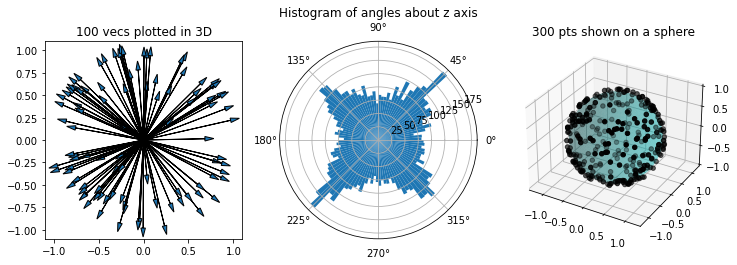There's a problem here; this method biases towards diagonal vectors. In the central plot, it seems like vectors near the axes are around 2/3rds as likely to be chosen as their diagonal counterparts. The difference is due to how these points are sampled - we take a random point in a cube and normalize it. There's far more 'space' along the diagonals, hence the skew.

A simple way to fix it is to ignore points in this 'extra space', repeating until we have a point inside the unit circle instead.

In :
def rand_vec1():
while True:
v = np.random.rand(3) * 2 - 1  # Random vec with values in range [-1, 1)
l = np.linalg.norm(v)          # Magnitude of v
if l <= 1:
v /= l                     # Random normal vector??
return v

vs = [rand_vec1() for i in range(10000)]
plot_3d_vecs(vs)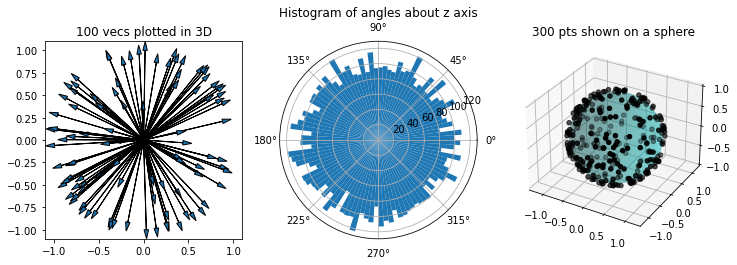Much better! There looks like an equal chance of 'choosing' a vector in any direction now. Still, the chance we discard a vector is $1 - \frac{4}{3}\pi0.5^2 \approx 0.47$. This means we'll often have to go through multiple iterations. Surely there's a better way...

If we use the values of the vector with a normal distribution, we can simply normalize to get a random vector. This is due to a neat property of the normal distribution - it's rotationally invariant.

In :
def rand_vec3():
v = normal(size=3)      # Random vec w/ normally distributed values.
v /= np.linalg.norm(v)  # Normalized = Random normal vector!?
return v

# Make a load of 'random' vectors.
vs = [rand_vec3() for i in range(10000)]
plot_3d_vecs(vs)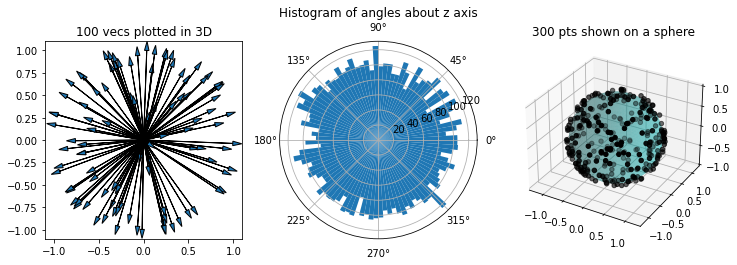## Uniform-random rotations¶

First, lets think about what a random rotation is. In 2 dimensions, rotations rotate an object by some angle from $0$ to $2\pi$, and it makes sense that a uniform-random rotation should be uniformly distributed over this range. In 3 dimensions/ euler angles this is no longer true though (as we'll see). We need a better definition.

Instead we can use the group-theoritic definition - that the distribution is unchanged by composition with arbitrary rotations.

In english (and very loosely) this is saying... Say we have a way to generate uniform-random rotations, and this has some distribution... If we apply any arbitrary rotation to these generated rotations, the distribution will remain the same.

For the sake of visualisation, we'll use a simpler 'sanity check'. A random rotation should rotate a fixed vector to point in any direction with equal probability. This is implied by the previous, when considering that a rotation can be represented by a basis.

### Euler angles¶

What happens if we just choose uniform-random elements of some euler angles?

In :
vs = []
for i in range(10000):
r = R.from_euler('zyx', np.random.rand(3)*2*np.pi) # Random rotation?
v = r.apply(np.array([0,1,0]))                     # Hence... Random vector?
vs.append(v)

plot_3d_vecs(vs)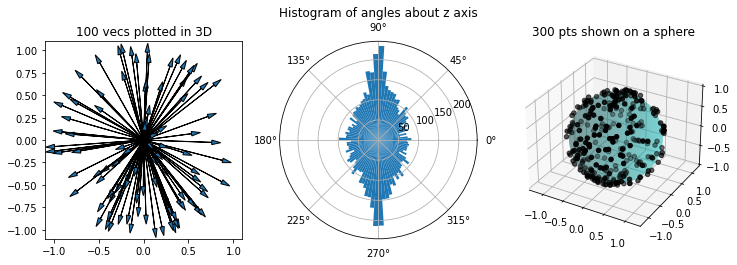Oh no! We can see that 'randomly' rotating an up vector overwhelmingly leaves aligned with the starting y axis. This is because of an effect called gimbal lock, and the order the euler angles are applied. In fact, it is possible to choose a uniform-random rotation from euler angles; choosing the angles according to a specific PDF (Miles, 1965)

$$g(\alpha, \beta, \gamma) = \frac{1}{8\pi^2} \sin \alpha, \;\;\; 0 \leq \alpha \leq \pi, 0 \leq \beta \lt 2\pi, 0 \leq \gamma \lt 2\pi$$

### Axis angle¶

Well we can create a random vector already... So is a uniform-random rotation just a rotation about a random axis by a random angle?

In :
vs = []
for i in range(10000):
ang = rand() * 2 * np.pi    # Random angle
vec = rand_vec1()           # Random vector
r = R.from_rotvec(ang*vec)  # = Random rotation?

v = r.apply(np.array([0,1,0]))  # Hence... Random vector?
vs.append(v)

plot_3d_vecs(vs)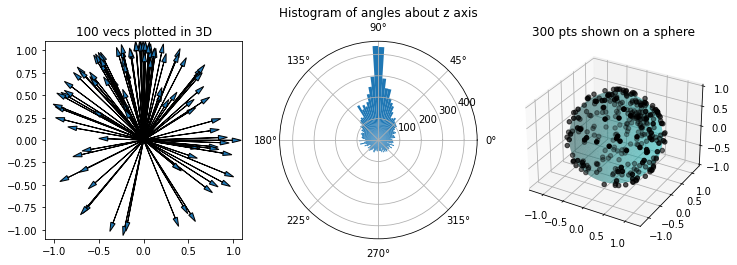Now the vectors are rarely changed far from their starting point?? Well, if the random axis chosen is close to the $y$ axis then the rotated vector will always remain close - regardless of the angle chosen. Again Miles has a PDF that corrects for this bias... But it's even more confusing than the previous. We define the rotation axis in terms of a tuple; $(\theta, \phi)$. These are effectively euler angles, placing a point on the surface of a unit sphere. And an angle $V$, about which to rotate. The PDF is then given by:

$$g(V, \theta, \phi) = \frac{1}{2\pi^2}\sin^2{\frac{1}{2}V\sin{\theta}}$$

Where $0 \leq V \leq \pi, 0 \leq \theta \leq \pi, 0 \leq \phi < 2\pi$

### Gram-Schmidt¶

A rotation in 3D can be expressed as a rotation matrix - which is simply an orthagonal normal basis. Since we can generate normal vectors, we can use the Gram-Shmidt process to create a random basis. Note that we must use Gram-Schmidt; SVD or qr decomposition alone do not produce an evenly distributed rotation.

A proof of the correctness of this approach is given in Multivariate statistics: a vector space approach, p234 (Eaton, 1983), or just verified below!

In :
def gram_schmidt(A):
for k in range(0, A.shape):
# Normalize
A[:, k] /= np.linalg.norm(A[:, k])
# Subtract from vecs below
for j in range(k+1, A.shape):
r = np.dot(A[:, k], A[:, j])
A[:, j] -= r*A[:, k]
return A

# Change the handedness of the matrix. Converts from S(3) to SO(3)
def make_left(A):
# If mat is right handed...
if np.cross(A, A).dot(A) < 0:
# Swap first two rows
A[[0,1]] = A[[1,0]]
return A

vs = []
for i in range(10000):
m = normal(size=(3,3))  # Random mat w/ normal distribution components
m = gram_schmidt(m)     # Random rotation mat?
#m = scipy.linalg.orth(m) # Uses SVD: Not uniform!

m = make_left(m)

# Simply apply rotation mat directly
v = m.dot(np.array([0,1,0])) # Hence, random vec?
vs.append(v)

plot_3d_vecs(vs)